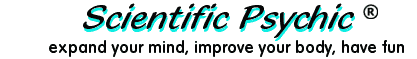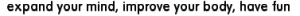IndexHow to code Square Root symbol in HTML, CSS and MathJax

Coding mathematical formulas in HTML is somewhat frustrating because HTML does not support formulas easily. It is possible to code simple mathematical equations with HTML markup without having to resort to using graphical images. The advantage of HTML and CSS over graphical images is that the display can be enlarged using the browser zoom or text-size options. When the Internet browser has JavaScript enabled, it is possible to use MathJax, a display engine for mathematics that works in all modern browsers.

A quadratic equation may be coded using the <sup></sup> tag.

ax2 + bx + c = 0

HTML Code:
ax<sup>2</sup> + bx + c = 0

HTML provides the square root character entity &radic; (√) which is a single character. In order to code the square root symbol, the characters that follow the square root character must have an overline. This is accomplished by creating a span of characters with a CSS style "text-decoration:overline;" as in the following example:

X + 1
HTML Code:
<span style="white-space: nowrap; font-size:larger">
</span>

Notice that the CSS style includes the nowrap option to keep the browser from splitting up the formula, and the formula within the square root has a leading and trailing non-blank space &nbsp; to extend the overline one character beyond the formula.

If you try to apply the above technique to a formula such as X2 + 1, the results don't look quite right because the overline applied to the superscript may be higher for some browsers, or it may cross over the superscripted characters:

X2 + 1         X2 + 1

Square Roots with superscripts may be coded using a table with two rows and two columns. The upper row contains &nbsp; to go over the square root symbol and enough underline characters to go over the formula. To avoid problems with rendering on different browsers, the table should use a widely availabe font family such as Verdana or Arial.

 _______ √ X2 + 1
HTML Code:
<table style="border-spacing:0px; border-width:0px; font-family:verdana;">
<tr>
<td>
&nbsp;
</td>
<td>
_______
</td>
</tr>
<tr>
</td>
&nbsp;X<sup>2</sup> + 1&nbsp;
</td>
</tr>
</table>

A technique that produces good results is to use a <span> tag, instead of the <sup> tag, to control the size and position of the superscripts so that they will not go into the overline. For example:

(a2.7+ b8.6
HTML Code:
(<i>a</i><span style="font-size: 10px;vertical-align:+25%;">2.7</span>+
<i>b</i><span style="font-size: 10px;vertical-align:+25%;">8.6</span>)&nbsp;</span>

The table technique may be expanded with additional rows to create quite complex expressions. The quadratic formula can be coded with a row where the overline in the denominator separates the numerator and denominator of the formula.

 −b ± √  b2 − 4ac 2a
HTML Code:
<table style="font-family:verdana;">
<tr>
<td>&minus;b &plusmn;
b<span style="font-size: 10px;vertical-align:+25%;">2</span> &minus;
4ac&nbsp;</span></td>
</tr>
<tr>
<td style="text-align:center;">
<span style="text-decoration:overline;">&nbsp; &nbsp; &nbsp; &nbsp; &nbsp;
2a &nbsp; &nbsp; &nbsp; &nbsp; &nbsp;</span>
</td>
</tr>
</table>

MathJax

MathJax is an open source JavaScript display engine for mathematics that works in all modern browsers. MathJax uses CSS and web fonts that make it possible to scale equations with surrounding text at all zoom levels. The documentation for MathJax is available at www.mathjax.org.

MathJax may be used in any web page by including the following JavaScript statement in the <head> section of the web page.

<script src="//cdn.mathjax.org/mathjax/latest/MathJax.js?config=TeX-AMS-MML_HTMLorMML"></script>

$x=−b±b2−4ac2a$

The Mathematical Markup Language (MML) defined by the W3C organization can be found here: www.w3.org/TR/MathML3/. The markup uses labels in XML format for operators, identifiers, numbers, etc. and defines their relationships. The markup document has an appendix that lists the codes for the mathematical operators at: www.w3.org/TR/MathML3/appendixc.html. In the example below, the code &#x2212; is used for the minus sign (−), &#x00B1; is used for the plus or minus sign (±), <mfrac> is used to define a fraction consisting of two rows for the numerator and denominator, and <msqrt> defines the contents within the square root radical.

<math display='block'>
<mrow>
<mi>x</mi><mo>=</mo>
<mfrac>
<!-- Start Numerator -->
<mrow><mo>&#x2212;</mo><mi>b</mi><mo>&#x00B1;</mo>
<msqrt>
<mrow>
<msup><mi>b</mi><mn>2</mn></msup><mo>&#x2212;</mo><mn>4</mn><mi>a</mi><mi>c</mi>
</mrow>
</msqrt>
</mrow>
<!-- Start Denominator -->
<mrow>
<mn>2</mn><mi>a</mi>
</mrow>
</mfrac>
</mrow>
[/itex]

Although the markup is complicated, the use of MathJax facilitates the display of mathematical equations without having to use graphic images.

Easy formulas using TeX and LaTeX

MathJax supports TeX, a typesetting system designed by Donald Knuth and released in 1978. A derivative product, LaTeX, was introduced in the early 1980s. Both TeX and LaTeX simplify the coding of formulas significantly. For example, the quadratic formula is coded within double dollar signs as follows:

$$x = {-b \pm \sqrt{b^2 - 4ac} \over 2a}$$

to generate the following:

$$x = {-b \pm \sqrt{b^2 - 4ac} \over 2a}$$

Documentation for LaTeX can be found at latex-project.org.